# Differentiation Techniques The Product and Quotient Rules 1

• Slides: 16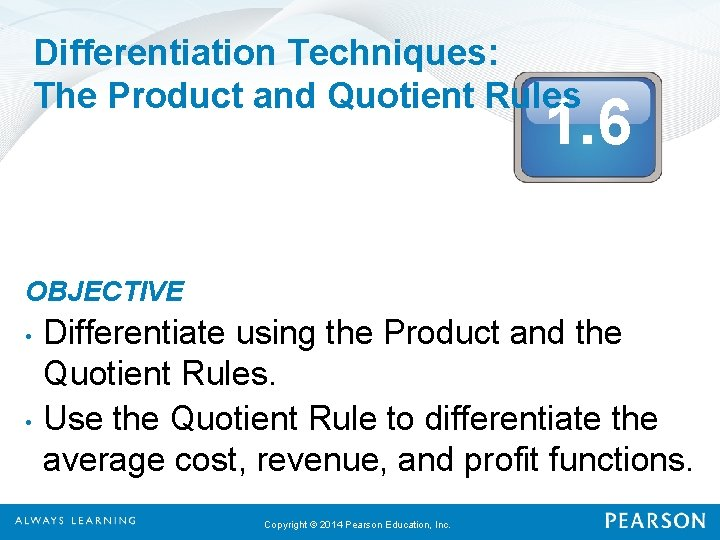Differentiation Techniques: The Product and Quotient Rules 1. 6 OBJECTIVE • • Differentiate using the Product and the Quotient Rules. Use the Quotient Rule to differentiate the average cost, revenue, and profit functions. Copyright © 2014 Pearson Education, Inc.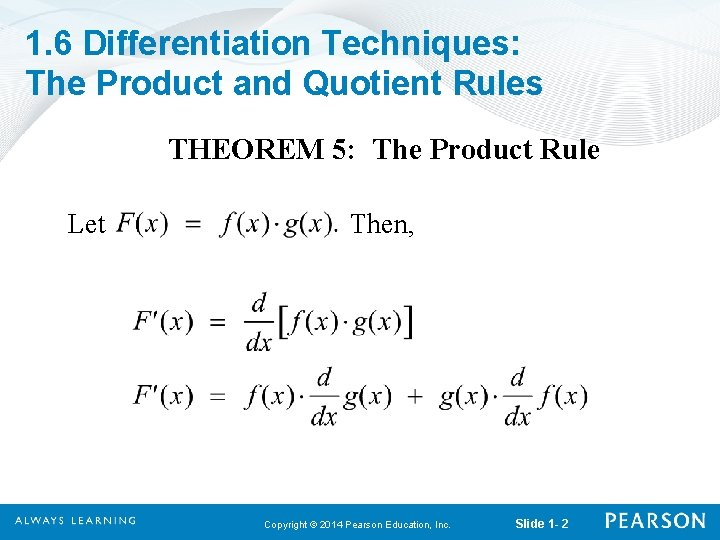1. 6 Differentiation Techniques: The Product and Quotient Rules THEOREM 5: The Product Rule Let Then, Copyright © 2014 Pearson Education, Inc. Slide 1 - 2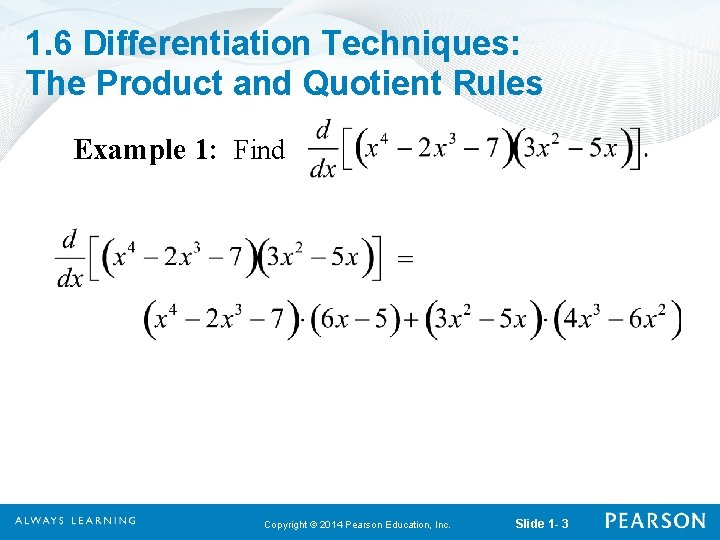1. 6 Differentiation Techniques: The Product and Quotient Rules Example 1: Find Copyright © 2014 Pearson Education, Inc. Slide 1 - 3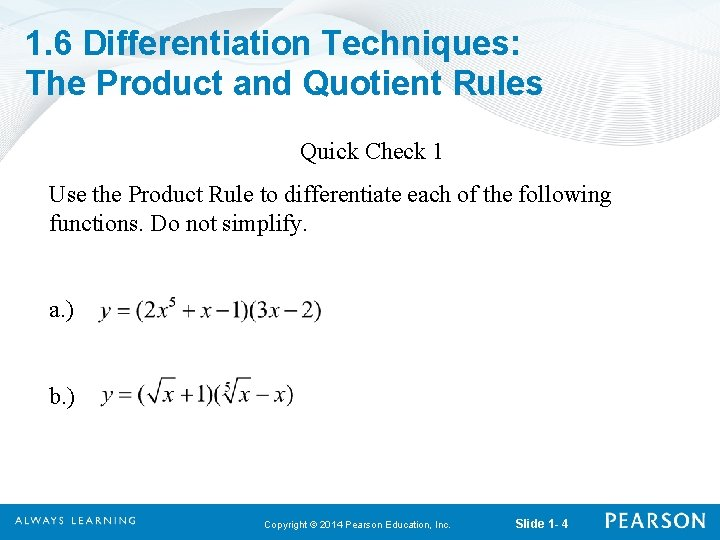1. 6 Differentiation Techniques: The Product and Quotient Rules Quick Check 1 Use the Product Rule to differentiate each of the following functions. Do not simplify. a. ) b. ) Copyright © 2014 Pearson Education, Inc. Slide 1 - 41. 6 Differentiation Techniques: The Product and Quotient Rules Quick Check 1 Solution a. ) Using the Product Rule: We get: b. ) Again, using the Product Rule, we get: Copyright © 2014 Pearson Education, Inc. Slide 1 - 5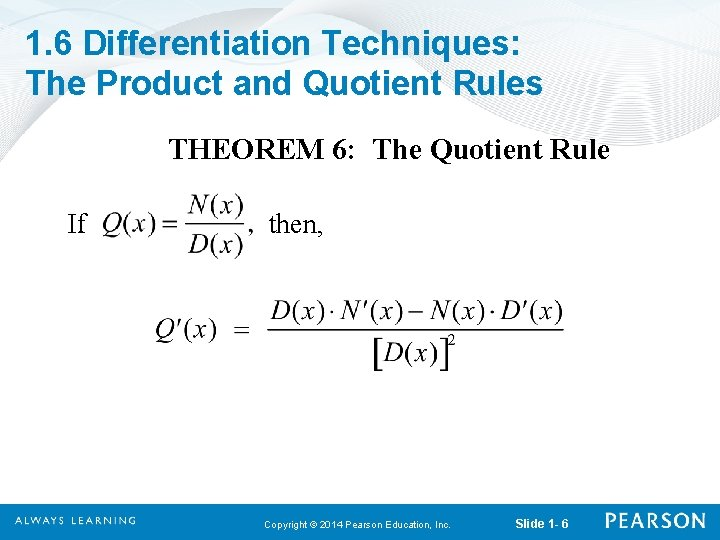1. 6 Differentiation Techniques: The Product and Quotient Rules THEOREM 6: The Quotient Rule If then, Copyright © 2014 Pearson Education, Inc. Slide 1 - 6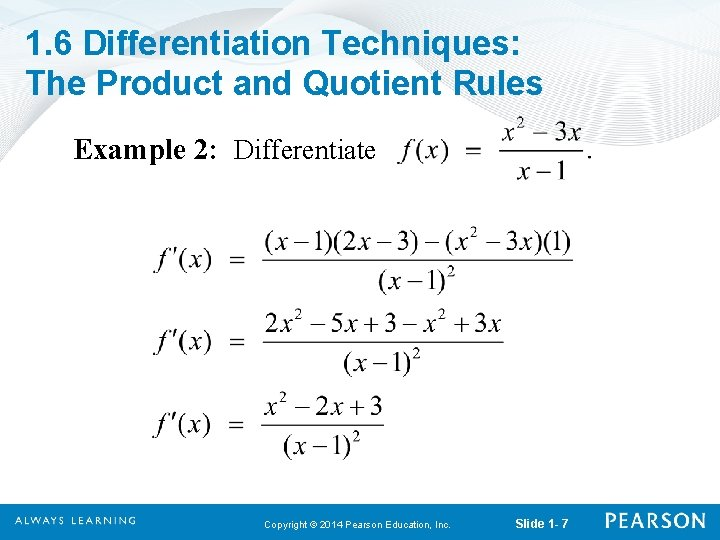1. 6 Differentiation Techniques: The Product and Quotient Rules Example 2: Differentiate Copyright © 2014 Pearson Education, Inc. Slide 1 - 7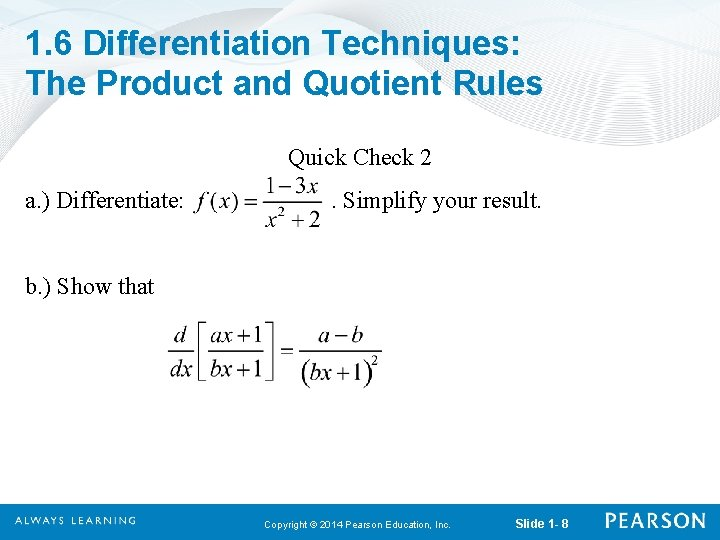1. 6 Differentiation Techniques: The Product and Quotient Rules Quick Check 2 a. ) Differentiate: . Simplify your result. b. ) Show that Copyright © 2014 Pearson Education, Inc. Slide 1 - 81. 6 Differentiation Techniques: The Product and Quotient Rules Quick Check 2 Solution a. ) Using the Quotient Rule: We get: Copyright © 2014 Pearson Education, Inc. Slide 1 - 9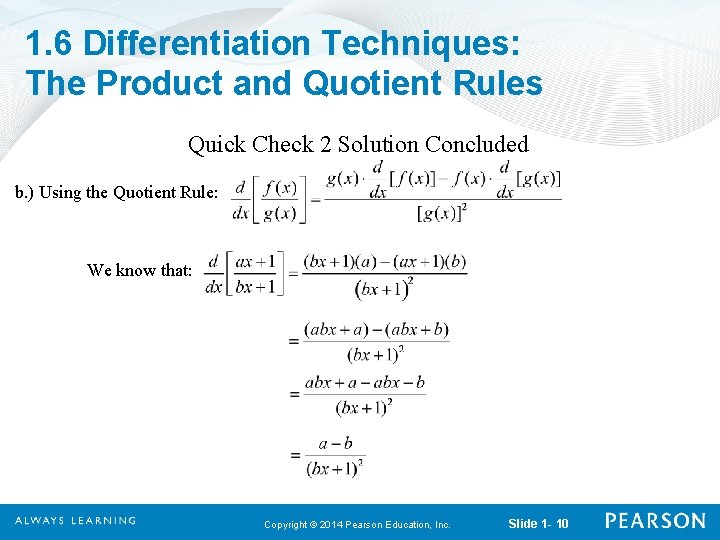1. 6 Differentiation Techniques: The Product and Quotient Rules Quick Check 2 Solution Concluded b. ) Using the Quotient Rule: We know that: Copyright © 2014 Pearson Education, Inc. Slide 1 - 101. 6 Differentiation Techniques: The Product and Quotient Rules DEFINITION: If C(x) is the cost of producing x items, then the average cost of producing x items is If R(x) is the revenue from the sale of x items, then the average revenue from selling x items is If P(x) is the profit from the sale of x items, then the average profit from selling x items is Copyright © 2014 Pearson Education, Inc. Slide 1 - 11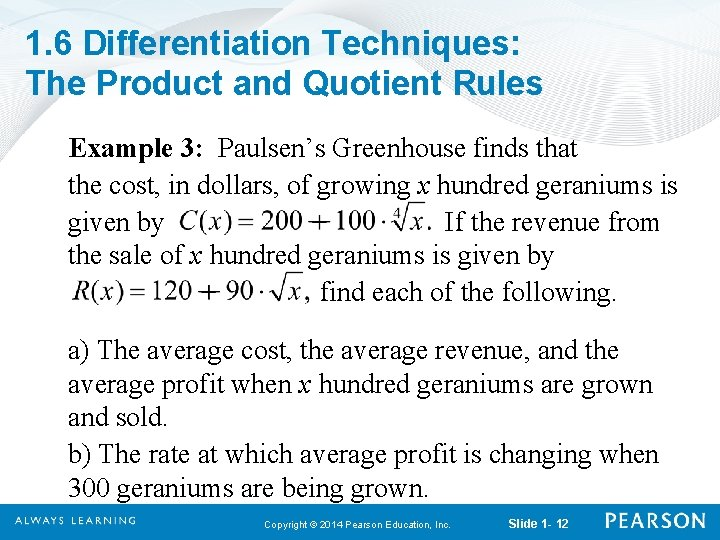1. 6 Differentiation Techniques: The Product and Quotient Rules Example 3: Paulsen’s Greenhouse finds that the cost, in dollars, of growing x hundred geraniums is given by If the revenue from the sale of x hundred geraniums is given by find each of the following. a) The average cost, the average revenue, and the average profit when x hundred geraniums are grown and sold. b) The rate at which average profit is changing when 300 geraniums are being grown. Copyright © 2014 Pearson Education, Inc. Slide 1 - 12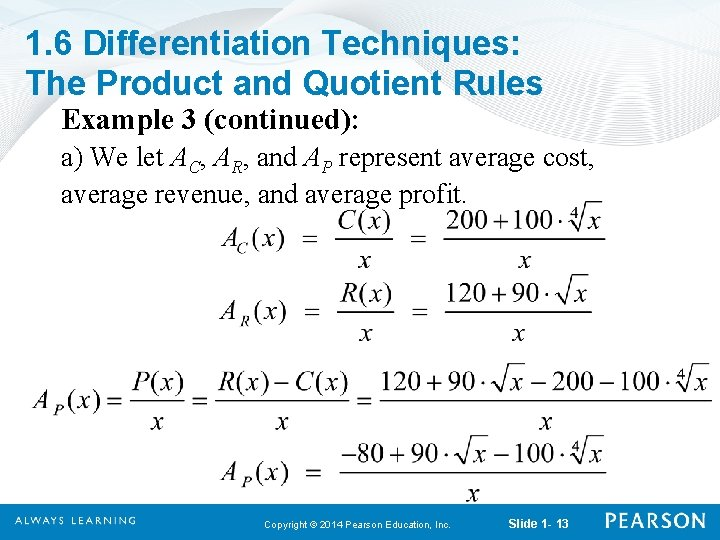1. 6 Differentiation Techniques: The Product and Quotient Rules Example 3 (continued): a) We let AC, AR, and AP represent average cost, average revenue, and average profit. Copyright © 2014 Pearson Education, Inc. Slide 1 - 13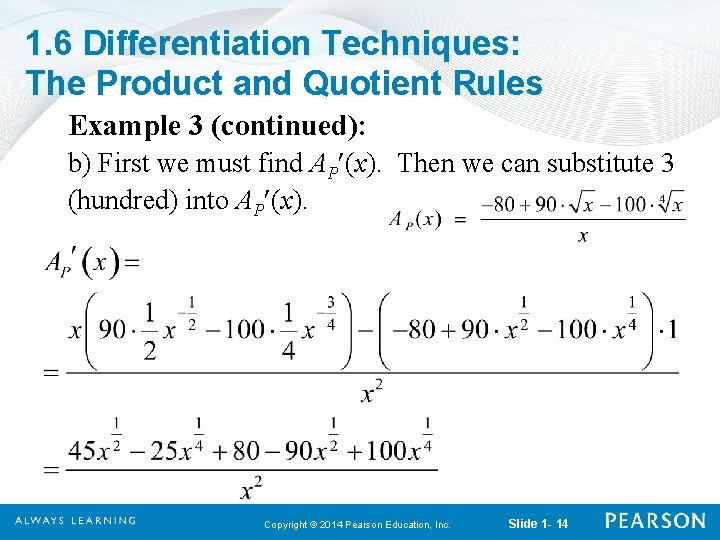1. 6 Differentiation Techniques: The Product and Quotient Rules Example 3 (continued): b) First we must find AP (x). Then we can substitute 3 (hundred) into AP (x). Copyright © 2014 Pearson Education, Inc. Slide 1 - 14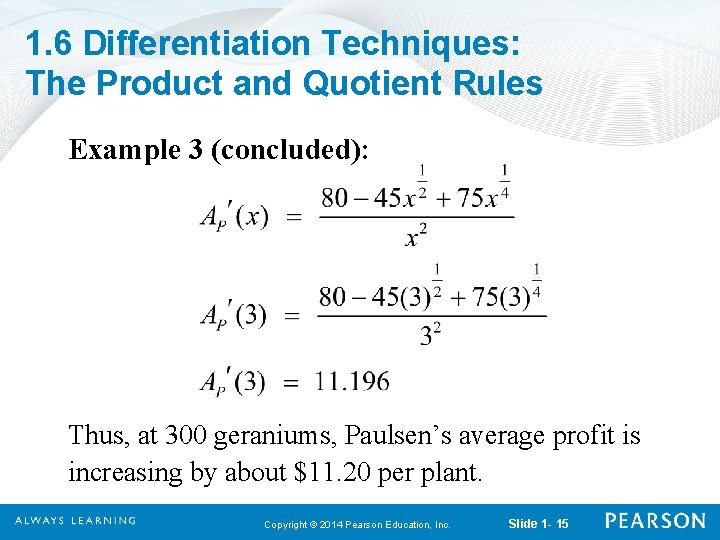1. 6 Differentiation Techniques: The Product and Quotient Rules Example 3 (concluded): Thus, at 300 geraniums, Paulsen’s average profit is increasing by about \$11. 20 per plant. Copyright © 2014 Pearson Education, Inc. Slide 1 - 15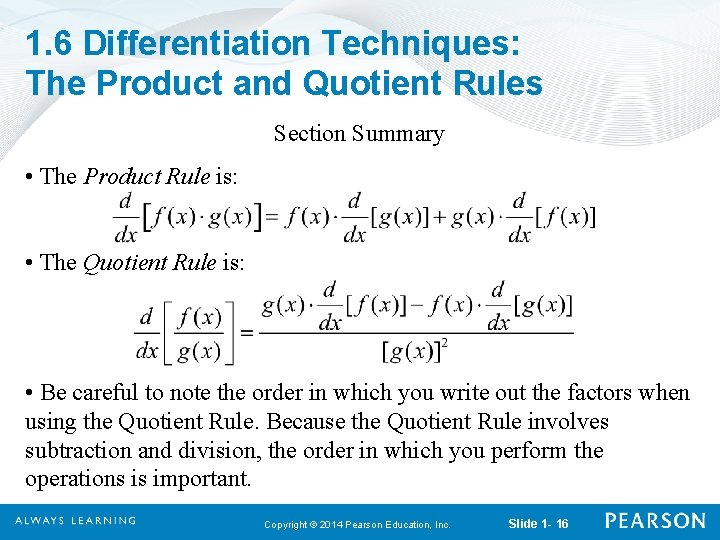1. 6 Differentiation Techniques: The Product and Quotient Rules Section Summary • The Product Rule is: • The Quotient Rule is: • Be careful to note the order in which you write out the factors when using the Quotient Rule. Because the Quotient Rule involves subtraction and division, the order in which you perform the operations is important. Copyright © 2014 Pearson Education, Inc. Slide 1 - 16